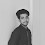# URI - BEECROWD - BEE 1067 - Odd Numbers Solution in C,C++,Python

## URI - BEECROWD - BEE 1067 - Odd Numbers Solution in C,C++,Python |  URI - BEECROWD - BEE Online Judge Solution 1067 - Odd Numbers

Read an integer value (1 <= <= 1000).  Then print the odd numbers from 1 to X, each one in a line, including X if is the case.

## Input

The input will be an integer value.

## Output

Print all odd values between 1 and X, including if is the case.

 Input Sample Output Sample 8 1357

### Demonstration:

Any integer that cannot be divided exactly by 2 is an odd number.
Here we apply a range-based for loop which provides all the integers available in the input interval.
After this, checking the condition for odd numbers are applied and printing these values.
N.B: Don't copy paste the code as same. Just try to understand it and try yourself.

### URI - BEECROWD - BEE Problem 1067 Solution in C :

 URI - BEECROWD - BEE Online Judge 1067 Solve  in C :
```#include<stdio.h>
int main()
{
int n,i;
scanf("%d",&n);
for(i=1;i<=n;i++){
if(i%2!=0){
printf("%d\n",i);
}
}
}
```

### 2 Responses to URI - BEECROWD - BEE 1067 - Odd Numbers Solution in C,C++,Python

1.here the problems condition is 1<=X<=1000

2.Yes.. start from 1 and instead of 1000,we take the input because we don't need to continue the loop 1000 times.. if we need to print odd numbers between 1 to 15,we don't need to continue loop after 15.